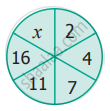Tamil Nadu Board of Secondary EducationSSLC (English Medium) Class 6th

# SSLC (English Medium) Class 6th - Tamil Nadu Board of Secondary Education Question Bank Solutions for Mathematics

Subjects
Topics
Subjects
Popular subjects
Topics
Mathematics
< prev  1 to 20 of 937  next >

The algebraic statement of ‘f decreased by 5’ is ____________

[0.012] Introduction to Algebra
Chapter: [0.012] Introduction to Algebra
Concept: Introduction to Algebra

The algebraic statement of ‘s divided by 5’ is ____________

[0.012] Introduction to Algebra
Chapter: [0.012] Introduction to Algebra
Concept: Introduction to Algebra

Draw the next two patterns and complete the table.Shapes 1st Pattern 2nd Pattern 3rd Pattern 4th Pattern 5th Pattern Squares 1 2 3 Circles 1 2 3 Triangles 2 4 6
[0.012] Introduction to Algebra
Chapter: [0.012] Introduction to Algebra
Concept: Introduction to Algebra

If ‘u’ is an even number, how would you represent the next even number?

[0.012] Introduction to Algebra
Chapter: [0.012] Introduction to Algebra
Concept: Introduction to Algebra

Express the following verbal statement to algebraic statement

[0.012] Introduction to Algebra
Chapter: [0.012] Introduction to Algebra
Concept: Introduction to Algebra

Express the following verbal statement to algebraic statement

4 times ‘q’

[0.012] Introduction to Algebra
Chapter: [0.012] Introduction to Algebra
Concept: Introduction to Algebra

Express the following verbal statement to algebraic statement

4 less to 9 times of ‘y’

[0.012] Introduction to Algebra
Chapter: [0.012] Introduction to Algebra
Concept: Introduction to Algebra

If ‘u’ is an even number, how would you represent the previous even number?

[0.012] Introduction to Algebra
Chapter: [0.012] Introduction to Algebra
Concept: Introduction to Algebra

Express the following algebraic statement to verbal statement

x ÷ 3

[0.012] Introduction to Algebra
Chapter: [0.012] Introduction to Algebra
Concept: Introduction to Algebra

Express the following algebraic statement to verbal statement

11 + 10x

[0.012] Introduction to Algebra
Chapter: [0.012] Introduction to Algebra
Concept: Introduction to Algebra

Express the following algebraic statement to verbal statement

70s

[0.012] Introduction to Algebra
Chapter: [0.012] Introduction to Algebra
Concept: Introduction to Algebra

The teacher asked two students to write the algebraic statement for the verbal statement “8 more than a number”. Vetri wrote ‘8 + x’ but Maran wrote ‘8x’. Who gave the correct answer?

[0.012] Introduction to Algebra
Chapter: [0.012] Introduction to Algebra
Concept: Introduction to Algebra

The number of days in ‘w’ weeks is

[0.012] Introduction to Algebra
Chapter: [0.012] Introduction to Algebra
Concept: Introduction to Algebra

The value of ‘x’ in the circle is[0.012] Introduction to Algebra
Chapter: [0.012] Introduction to Algebra
Concept: Introduction to Algebra

The value of ‘y’ in y + 7 = 13 is

[0.012] Introduction to Algebra
Chapter: [0.012] Introduction to Algebra
Concept: Introduction to Algebra

6 less to ‘n’ gives 8 is represented as

[0.012] Introduction to Algebra
Chapter: [0.012] Introduction to Algebra
Concept: Introduction to Algebra

Complete the following pattern.
9 – 1 =
98 – 21 =
987 – 321 =
9876 – 4321 =
98765 – 54321 =
What comes next?

[0.012] Introduction to Algebra
Chapter: [0.012] Introduction to Algebra
Concept: Introduction to Algebra

Ratio of ₹ 3 to ₹ 5 = ______________

[0.013000000000000001] Ratio and Proportion
Chapter: [0.013000000000000001] Ratio and Proportion
Concept: Concept of Ratio

Ratio of 3 m to 200 cm = _____________

[0.013000000000000001] Ratio and Proportion
Chapter: [0.013000000000000001] Ratio and Proportion
Concept: Concept of Ratio

Ratio of 5 km 400 m to 6 km = _______________

[0.013000000000000001] Ratio and Proportion
Chapter: [0.013000000000000001] Ratio and Proportion
Concept: Concept of Ratio
< prev  1 to 20 of 937  next >